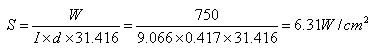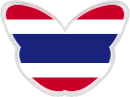## ติดต่อ Alloy Wire

120/55 ซอยสุขุมวิท 101/1 บางจาก พระโขนง จ.กรุงเทพฯ 10260

โทร: +66 (0) 80 626 9989
แฟกซ์: +66 (2) 398 6391
อีเมล: chompoonuut_t@alloywire.com# Designing a Round Wire ส่วนประกอบ

Where:

 V Voltage (Volts) W Power (Watts) S Surface Area Loading (W/cm2) Rt ส่วนประกอบ Resistance at Operating อุณหภูมิ (ohms) R ส่วนประกอบ Resistance at 20°C (ohms) F อุณหภูมิ-Resistance Factor I Wire Length (m)

Example:

1.  To calculate the wire diameter and length required for a 750w/240v pencil bar fire element, operating at a maximum temperature of 1100°C, the total resistance of the element at operating temperature (Rt) will be: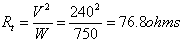2.  Using RW80 wire, the อุณหภูมิ Resistance Factor (F) at 1100°C is 1.071 thus the total resistance of the element at 20°C (R) will be: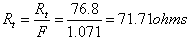3.  Knowing the dimensions of the pencil bar, the length of wire that may be wound round it may be estimated (see coiled or spiral elements).  Thus, the resistance required per metre of wire will be:

For example a length of wire of 9 metres –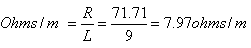4.  RW80 of wire diameter 0.417mm has a resistance per metre of 7.91 ohms/m (see stock sizes page) which is closest to 7.97 ohms/m.

5.  To verify the actual wire length (L):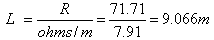A change in wire length may mean adding or subtracting turns (altering the pitch of the wire) to achieve the total resistance value required.